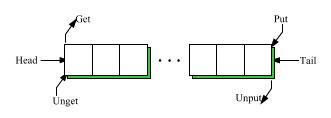DOC HOME SITE MAP MAN PAGES GNU INFO SEARCH PRINT BOOK
A List Class Library for C++ - List(C++)

# Queue-oriented operations

This group of functions treats a List as a queue. The primary operators are `put()` and `get()`, which correspond to `<<` and `>>` on streams. We also supply `unput()` and `unget()`, which make it possible to use a List as a stack or deque. In addition, `head()` and `tail()` can be used to examine the elements at the beginning and at the end of the List without removing them. Figure 1 shows the relationships of the queue-oriented functions. They allow new Ts to be added or removed at either end of the `List<T>`. `put()` and `unget()`, the operators that add Ts to the List, can also take `List<T>` arguments, so they serve as append and prepend operators (correspondingly) as well.Queue-oriented functions

`L.head()` returns a pointer to the element at the head of the List L. If the List is empty, zero will be returned.

## Tail

`L.tail()` returns a pointer to the element at the tail of the List L. If the List is empty, zero will be returned.

## Put

`L.put(t)` puts T `t` on the end of List `L` and `L.put(l)` appends List `l` to `L`. `put()` returns its updated object so `L.put(t1).put(t2)` will append `t1` and `t2` to `L`.

## Get

If `L` is non-empty, `L.get(t)` gets (and removes) the first element from `L`, assigns it to `t`, and returns TRUE. Otherwise, `L` and `t` are unchanged, and the return value is FALSE.

## Unget

`L.unget(t)` undoes the effect of `L.get(t)` (which removes the first character from `L`); that is, it puts `t` back on the front (got that?) of `L`. In another sense, `unget()` is the reverse of `put()` since it prepends rather than appends to its object. The type rules are exactly the same as for `put()`. `unget()` returns its object.

## Unput

`L.unput(t)` undoes the effect of `L.put(t)` (which appends `t` to `L`); that is, it gets (and removes) the last element from `L`, and assigns it to `t`. Similarly, in another sense, `unput()` is the reverse of `get()` since it removes the last (instead of the first) element of its List.

Versions of `get()` and `unput()` exist that do not take an argument. They remove an element from the List and return it, if the List is non-empty. If the List is empty, this is considered a program bug and the result may be unexpected (see "Error handling" below). It is the application's responsibility to make sure that the List is not empty.

The original `remove()` function at the beginning of the paper is an example of the use of `put()` and `get()`. A stack can be easily constructed using `put()` and `unput()` in the following way:

```   ```class int_stack : List<int>
{
public:
int_stack() {}
~int_stack() {}
int   empty()   { return length() == 0; }
int   pop()     { int x; if (!unput(x)) error();
else return x; }
void  push(int x) { put(x); }
}; ```
```

Clearly, `unget()` and `get()` could have been used instead of `put()` and `unput()`.

Next topic: Element selection
Previous topic: Sort operation